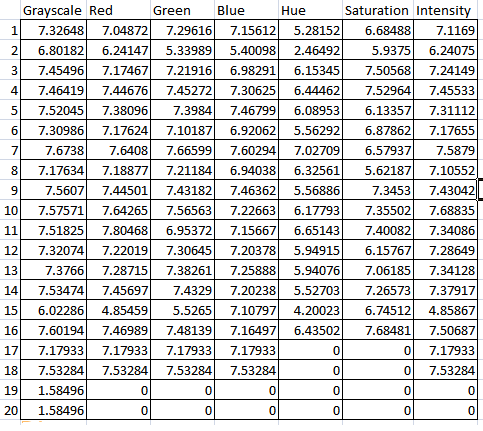In the past, I have always encountered these questions while doing my research. To truly emulate human vision perception in computer vision, I need to understand how the mechanism works. These questions form the fundamental mechanism of human vision. Therefore, I decided to compute several images to proof my believe. My main hypothesis is human vision is represented in HSV colour model rather than RGB colour model. To proof this, I will be using basic Information Theory to compute the information composition in an single image both RGB and HSV.

### Information Theory#

$$\begin{equation} H(X) = -\sum_{x \in \mathcal{X}} p(x) \log_b p(x). \end{equation}$$

###### Shannon’s Entropy Equation#

The basic Shannon Entropy will be used to compute each individual plane in an image. This entropy is used to measure the unpredictability. The easier to predict, the lower the entropy it has. In this case, I also made an assumption that entropy directly relate to the information contained / encoded within. If you need detail description on Shannon Entropy, head over to http://en.wikipedia.org/wiki/Entropy_(information_theory) which contains fairly understandable explanation.

### Methods#

A set of 20 various images from grayscale to single colour, from human and non-human images are collected. Entropy will be computed on grayscale mode, Red plane, Blue plane, Green plane as well as Hue plane, Saturation plane and Intensity.

### Results and Explanation#As expected RGB plane has pretty similar entropy in every images. If one of the plane has low entropy, both the plane will follow including the grayscale version of the image. On the other hand, HSV has a pretty interesting results. Averagely, Intensity plane has higher entropy than the other Hue and Saturation. In the event of grayscale image (note Image 17 and 18), Intensity contain entropy but not the other two.

### Conclusion#

1. Intensity planes contain more information. Therefore, we are able to perceive an image from only the intensity plane.
2. Single colour image does has information but when converted to HSV plane, it represents 0 entropy in every plane.
3. Human vision is closer to HSV than RGB. Notes: Cone cell in retina
1. Wikipedia on Shannon Entropy http://en.wikipedia.org/wiki/Entropy_(information_theory)
2. Beginner explanation of how entropy is applied in classification http://stackoverflow.com/questions/1859554/what-is-entropy-and-information-gain
3. Kodak Lossless Image Suite used in Sample Images http://r0k.us/graphics/kodak/